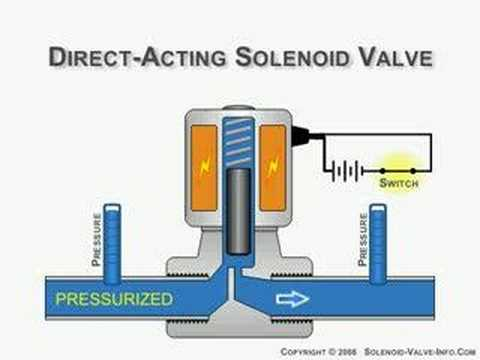# Solenoid valve pressure drop

I'm designing a solenoid valve for an automated irrigation project that I'm working on. After having looked at some designs, I have a question.For most of the designs I've seen, the orifice is smaller than the pipe diameter. Wouldn't this reduce the flow rate? If this is the case and if I'm using such a design, do I make that opening bigger?

A valve that is smaller than the pipe diameter will reduce the flow rate, but so will any valve. When calculating what sort of pressure/head loss (pressure and head are equivalent) a system will have, there are two contributing factors: major losses and minor losses.

$$h_{total}=h_{major}+h_{minor}$$ $$h_{major}=\lambda \left( \frac{l}{d_h} \right) \left( \frac{v^2}{2g} \right)$$ $$h_{minor}=\xi \left( \frac{v^2}{2g} \right)$$

Because these losses are expressed as head, they are in units of length. $\lambda$ is the friction factor, which is a complex number that varies based on the type of flow (laminar, turbulent, transitional) and then the Reynolds number and tube roughness. That's a topic worth exploring elsewhere, but isn't directly related to your question. $l$ is the length of straight pipe, $d_h$ is the hydraulic diameter, which for a circular pipe is the actual diameter. As pipe shape changes, there are different expressions for hydraulic diameter. $v$ and $g$ are flow velocity and gravitational acceleration, as you would expect.

The second equation is the one we're really looking at though. This is how you calculate the head loss across the valve. $\xi$ is the loss coefficient, and $v$ and $g$ are the same as above. The only way you can get loss coefficients is by looking them up or by calculating them empirically, if values don't exist for your components.

We also have to account for the change in overall diameter of the pipe though. This can be done a couple ways, depending on how the pipe diameter changes. If it's sudden, it's probably best to treat it as another pipe feature which will cause a pressure drop.

In a more gradual change, you will have some head loss, but the bigger effect will be the narrowing diameter increasing the velocity of the flow. This is a fairly trivial result of conservation of mass. The mass flow rate in and out must be the same, and if we assume constant density (reasonable for liquid water at speeds under 450 m/s {1000 mph}), then we'll see that velocity varies inversely with cross-sectional area.

$$\dot{m}_{1}=\dot{m}_{2}$$ $$\dot{m}=\rho \dot{V}$$ $$\dot{V}=Av$$ $$\therefore A_{1} v_{1}=A_{2} v_{2}$$

This $v_{2}$ is the inlet velocity of the valve, and if the velocity increases, so will the minor loss across the valve. So yes, increasing the diameter of the valve will make the pressure drop go down, but you can't eliminate the pressure drop entirely.

Ultimately, standard designs are standard for a reason. The valves constrict a bit, but they don't bulge out more than necessary from the rest of the pipe.

Most equations referenced from Engineering Toolbox

• wow. that was such a good explanation. thanks :) So is there any way to compensate for this loss? What I don't seem to understand is how the standard solenoid valves work, as they all seem to have a smaller orifice with the valve. Also, does the smaller orifice within the valve increase the flow rate hence compensating for the diameter differences? – azhamn May 17 '15 at 2:56
• Usually the way we go about pipe system design is to determine what sort of conditions we want to achieve at certain points in the system (flow speed, mass flow rate, pressure). Then, knowing various inputs (inlet pressure if it's not a loop, pump capability, etc.) we can use the pressure loss across the system to determine what kind of pumping power we need. That's a fairly in-depth topic though, it's a big chunk of an introductory fluids course. But the compensation is essentially picking a pump that works with the losses in the system. – Trevor Archibald May 17 '15 at 3:03
• Oh ok. Seems like I've gotten myself into something too complex for me. In this particular case, there is no pump. The water is from a tank which is roughly 50 feet above ground. I think i'll get a prototype done and experiment. no better way to learn! thanks @Trevor Archibald – azhamn May 17 '15 at 20:19

The total flow resistance is the sum of its parts. Pipes are long, valves are short. Making a pipe wider is relatively inexpensive, and making a valve wider is relatively expensive. As you make the opening wider, everything about the valve has to be beefed up, as the forces involved will scale along with the size of the opening. So on balance, youd always expect valve openings to be smaller relative to pipe dimensions, in a cost optimized system.